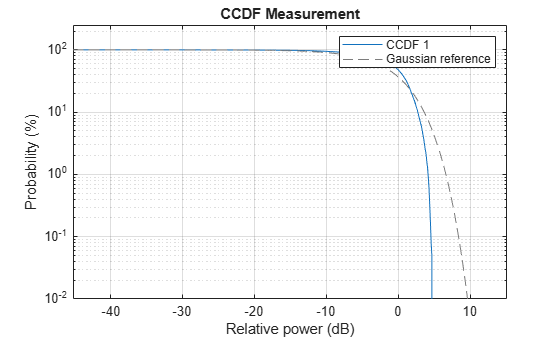# relativePower

Use CCDF to find relative power for specified probability

Since R2022a

## Syntax

``relpower = relativePower(meter,prob)``

## Description

example

````relpower = relativePower(meter,prob)` computes the relative power `relpower` for a specified probability `prob`, such that the instantaneous signal power is above the average signal power by `relpower` dB with probability `prob`/100. To enable this syntax, set the `computeCCDF` property to `true` in the `powermeter` System object™.```

## Examples

collapse all

Compute the average power measurement of the generated voltage signal.

```x = complex(rand(10000,1)-0.5,rand(10000,1)-0.5); pm = powermeter(ComputeCCDF=true); averagePower = pm(x); % power in dB```

Compute the probability that the instantaneous power of the signal is 3 dB above the average power.

`prob = probability(pm,3) % probability in percentage`
```prob = 7.6009 ```

Plot the CCDF curve.

`plotCCDF(pm,GaussianReference=true)`Find the relative power for the given probability.

`relativepwr = relativePower(pm,prob)`
```relativepwr = 3.0000 ```

## Input Arguments

collapse all

Power meter, specified as a `powermeter` System object.

Probability in percentage, specified as a nonnegative column vector. The probability that the instantaneous signal power of the jth channel is above its average signal power by `relpower`(i,j), in dB, is `prob`(i)/100.

If the `PowerRange` property is not set sufficiently large, the computed CCDF curve may not reach some probability values in `prob` and the corresponding values in `relpower` is `NaN`. If no `prob` value is provided, `relativePower` returns `relpower` for `prob` = [10 1 0.1 0.01 0.001 0.0001]'.

Data Types: `single` | `double`

## Output Arguments

collapse all

Relative power in dB, returned as an N-by-M matrix. N equals the number of rows in `prob` and M equals the number of signals provided.

Data Types: `double`

## Version History

Introduced in R2022a